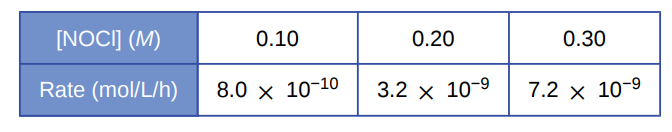# Problem: Nitrosyl chloride, NOCl, decomposes to NO and Cl2.2NOCl(g) ⟶ 2NO(g) + Cl2 (g)Determine the rate equation, the rate constant, and the overall order for this reaction from the following data:

###### FREE Expert Solution

Determine the rate equation, the rate constant, and the overall order for this reaction

2NOCl(g) ⟶ 2NO(g) + Cl2 (g)

Step 1. Calculate the order

2 raised to 2 = 4

x = 2 → 2nd order

Since there is only 1 reactant, the overall order is 2nd order

Step 2. Setup rate law

The rate law of the reaction is: rate =k[NOCl]2

92% (129 ratings)###### Problem Details

Nitrosyl chloride, NOCl, decomposes to NO and Cl2.

2NOCl(g) ⟶ 2NO(g) + Cl2 (g)

Determine the rate equation, the rate constant, and the overall order for this reaction from the following data:Frequently Asked Questions

What scientific concept do you need to know in order to solve this problem?

Our tutors have indicated that to solve this problem you will need to apply the Rate Law concept. You can view video lessons to learn Rate Law. Or if you need more Rate Law practice, you can also practice Rate Law practice problems.

What professor is this problem relevant for?

Based on our data, we think this problem is relevant for Professor Combrink's class at TAMIU.

What textbook is this problem found in?

Our data indicates that this problem or a close variation was asked in Chemistry - OpenStax 2015th Edition. You can also practice Chemistry - OpenStax 2015th Edition practice problems.